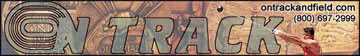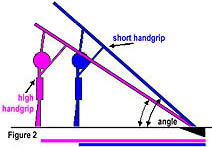Physics of the Pole Vault

by Kimo Morris

I am not an expert in physics. Fortunately, the general concepts of pole vault physics are rather simple. Here, I present a VERY simple model with which to have fun. As an example of how any average vaulter can figure out their maximum potential height, we can do some simple math to get a ball park figure. The basic idea is this - a pole vaulter takes his/her horizontal acceleration and converts it into vertical acceleration (counteracting the acceleration of gravity) through the use of a compressible spring (that would be the pole). To derive an equation, one must first make a few assumptions to start. Okay, so you may recall that FORCE equals MASS times ACCELERATION (good ole' Newton's f=ma equation). In the case of the vault, this means that the amount of force applied to the pole at the plant equals the mass of the vaulter (his/her weight) times the vaulter's acceleration at the plant (where acceleration is the measure of change in the velocity or speed). So the first assumption we will make is that the "energy" at the bottom of the plant is converted to vertical lift without any loss. This of course does not happen in real life. No vaulter can convert 100% of the energy created at the plant into vertical lift. Much of it is lost in pole vibration, dissipation in the fiberglass, technique, etc. However, by making this assumption, we can make a mathematical model to figure out what the absolute highest height would be for a vaulter with a given weight and speed. So, we will start our model by saying that 100% of the KINETIC ENERGY gets converted to POTENTIAL ENERGY. From your intro to physics book, you know that the equation for calculating potential energy as one increases their height off the ground is:

PE = mgx

where m is mass (calculated from weight), g is the acceleration of gravity, and x is the height off the ground. Again, from your physics book, you can also get the equation for calculation Kinetic Energy:

KE = 1/2 mv2

where m is mass, and v is velocity (which is the same as speed in our equation). Since we've made the assumption that all the kinetic energy will equal the potential energy, you can just make the equations equal to each other like this:

mgx = 1/2 mv2

If you solve for x (height), you get

x = (1/2 v2)/g

This solution says a lot! First, notice that mass no longer appears in the equation. In fact, there are only two things left in the equation - speed and gravity. Obviously, gravity is constant. Thus, your absolute height will be determined by how fast you run! Now, let's come back down to reality. There is a lot more to pole vaulting than just running fast. One must work on proper technique throughout the entirety of the vault. The better your technique, the less energy you will lose throughout the vault. But, a generality can be made here. Assuming it were possible for two vaulters to have the exact same technical skills, the one that jumps the highest is the one that can run the fastest. So, let's get back to our equation. Suppose you can run a 100m race in 12 seconds. Your speed (velocity) would therefore be 100m/12s = 8.33 m/s. Gravity is 9.8 m/s2. Plugging this into the equation and you get:

x = (1/2 v2)/g = (1/2 (8.33m/s)2)/9.8m/s2 = 3.54m which is the same as 11'8"

But wait a minute! That does not seem that high. Remember, this is just a mathematical model. Our little equation does not know the difference between a ball of lead and a water balloon, let alone the shape of a human body. It simply calculates a distance based on the center of mass of an object. From a math standpoint, our pole vaulter is just a little sphere with a center of mass in the middle. The fact is, the center of mass of a normal human adult is around 3' 6" off the ground. So, our estimate of height means that your center of mass at the end of the vault will be 11' 8" above the center of mass when you are still on the ground. Thus, you must add 3' 6" and 11' 8" to get 15' 2". At this point, again, we must bring reality back into it. By getting completely inverted and pushing off the top of the pole, a vaulter can actually "pull" and then "push" their center of mass higher as the pole uncoils (unbends). See my UCI picture from 1994 to get a visual idea of this. Really good vaulters can push their hips (center of mass) up as much as 4' beyond their top hand grip.

Clearly, there is much more to the vault. Other important variables come into play. To truly understand the vault from an advanced perspective, one must understand how the vault is affected by things like the TAKE OFF ANGLE.This is roughly determined by the angle the pole makes with the ground at the plant when your right arm (for right-handed vaulters) is straight above your head. If two vaulters hold in the exact same place on a pole, but one vaulter is taller, then the taller vaulter will create a larger angle at takeoff, and will subsequently be closer to the box (Figure 1). The shorter vaulter will have to compensate by either running faster, or modifying their takeoff to give the effect of having the same angle at takeoff of the taller vaulter. This is why coaches constantly tell their vaulters to take shorter, quicker strides at the end of the run, so the center of mass is as high as possible at the take off. Another technique used by more advanced vaulters is "jumping into" the vault, that is, beginning the long-jump-style take off before the bottom of the pole has contacted the box. What advanced vaulters do to accomplish this is to jump outside of their standard take off, also known as the plumb line (plumb line is defined as the place where your take off toe touches the runway when you are standing perfectly straight with the pole in the box and your arms extended above your head without leaning backward or forward - we all do this when we check our step). This is known as "taking off outside". I DO NOT recommend doing this unless you are experienced. If you do not have enough speed at the plant, taking off outside could lead to serious problems. Only attempt taking off outside under the instruction of an experienced coach.The height of ones hand grip will also effect the angle at take off. If we have two vaulters of identical height, but one vaulter holds higher (closer to the top end of the pole), then the vaulter who holds higher will create a smaller angle at take off (Figure 2). This means that as you improve and you begin to hold higher on the pole, you will have to compensate by running faster, keeping your center of mass high, and producing more force at the plant.

Along with the angle of take off, the distance of ones take off from the box also affects the vault. Since the distance of take off is related to the angle of take off, it is hard to see why they are separate things. However, think of it this way. The further away from the box you are, the greater the distance that you must carry your energy to complete the vault. Again, the shorter you are, or the higher you hold, the greater your take off distance will be from the box. Speed at the plant is key for this variable. Let's face it, holding higher means you have to run faster and hit the back of the box harder!

For more on the trade offs of the pole vault, I suggest checking out the paper by Nicholas P. Linthorne of the Department of Sport Science, Brunel University, UK. He ran computer models of the trade offs mentioned above (among other things) to come up with the theoretical optimal combination. His publication appears in Sports Engineering 3(4): 205-218 (2000).
 Download the paper here [pdf]. DISCLAIMER: This paper is published by Blackwell Science, LTD. You may download this paper if you are a student or faculty member of a qualifying institution and will use the paper for research or instructional purposes ONLY.

To learn more about the mechanics of the vault, I have listed a few websites below that will help. Please send me feedback and let me know if this page was useful to you. Happy vaulting! - Kimo